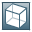Show TOC

###Interest Calculation MethodsThis interest calculation method is a day count convention (procedure for counting days) for interest calculation . It specifies how a part of the year between two calendar dates is determined.

The interest calculation method leads from percentage calculation to percentage calculation for a specific period.

The interest calculation method consists of the quotient of for the day method and the base day method.

These methods define how you determine the number of days and base days that are elements of the formulas for linear interest calculation and exponential interest calculation.

#### Structure

You can use the following interest calculation methods:

• 360E/360

• 360E/365

• 360E / actY

• 360 / 360 (German)

• 360 / 365 (ISDA)

The abbreviation ISDA stands for International Swaps and Derivatives Association.

• 365 / 365 (ISDA)

• m+act / 360

• m+30 / 360

• 360 / 360

• 365 / 360

• 365 / 365

• act / 360

• act / 364

• act / 365

• act / 366

• act / actY (ISDA)

• act / actP (ICMA)

The abbreviation ICMA stands for International Capital Market Association.

• act / actE (AFB)

The abbreviation AFB stands for Association Française des Banques (Association of French Banks).

• actW / 252

#### IntegrationThe following figure shows how to use the interest calculation method in interest calculation. The system changes the interest calculation method into the day method and the base day method. These methods define how days and base days are determined for the interest calculation period.